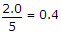# Electronics and Communication Engineering - Microprocessors

### Exercise :: Microprocessors - Section 4

16.

Consider the following expressions in Java
`int a, b, c, a = 40;b = a++ ;c = ++a ;`
Now the values of a, b, c are

 A. 42, 40, 42 respectively B. 42, 42, 42 respectively C. 40, 42, 42 respectively D. 40, 40, 42 respectively

Explanation:

The steps are : a is given the value 40, b is given the value of a before it is incremented (i.e., 40) and a is incremented to 41, a is incremented to 42 and c is given this value. Thus the result is 42, 40, 42.

17.

Which of the following is not correct in C?

 A. 6 / 4 = 1 B. 2 / 5 = 0 C. 2.0 / 5 = 0 D. 2.0 / 5.0 = 0.4

Explanation:18.

Which is not a software?

 A. DOS B. Windows C. MS WORD D. Hard disk

Explanation:

Hard disc is a memory storage device.

19.

Which of the following integer expressions is incorrect in Pascal?

 A. `L div M + P ` B. `A * * B` C. `A div B - C ` D. `A mod B`

Explanation:

Two operators cannot be together.

20.

Accumulator is an 8 bit register and is also known as register B.

 A. True B. False